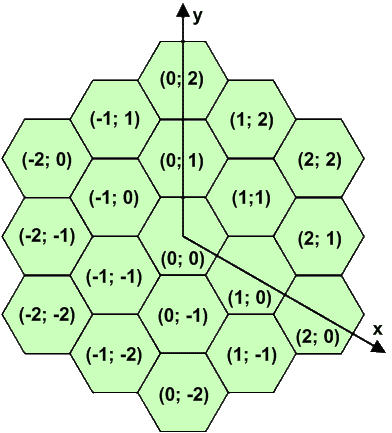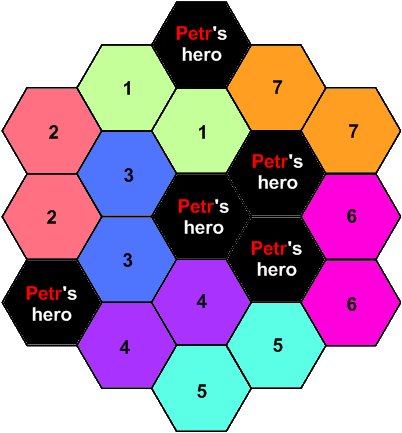### Problem Statement

You may have heard of the game "Heroes of Might and Magic". Recently, battles in this game have been broadcast online, and the weekly battles between Petr and Vasyl have been very popular. Petr usually wins these battles, so Vasyl has decided to develop a new strategy.

The battlefield in this game consists of equal hexagonal cells arranged in the form of a larger hexagon. The number of cells on each side of the battlefield represents the size of the field. The coordinate system is shown in the following figure, which depicts a battlefield of size 3:This week, Petr and Vasyl are playing on a battlefield of size N. Some of the cells are already occupied by Petr's heroes. The locations of these cells are given in the int[]s X and Y, where (X[i], Y[i]) is the location of Petr's i-th hero. To defeat Petr, Vasyl must occupy every single free cell with his own heroes. Unfortunately, Vasyl only has equestrian heroes, each of which occupy exactly two adjacent cells. No two heroes can occupy a single cell. One possible correct arrangement is shown in the following figure:Vasyl wants to simulate a battle with every possible arrangement of his heroes so that he can determine the optimal one. Calculate the number of different possible arrangements he will consider. The answer might be very large, so return the number modulo 2000000011.

### Definition

 Class: HexagonalBattlefield Method: countArrangements Parameters: int[], int[], int Returns: int Method signature: int countArrangements(int[] X, int[] Y, int N) (be sure your method is public)

### Constraints

-N will be between 1 and 8, inclusive.
-X will contain between 1 and 50 elements, inclusive.
-X and Y will each contain the same number of elements.
-All points (X[i], Y[i]) will lie inside the battlefield of size N.
-All points (X[i], Y[i]) will be distinct.

### Examples

0)

 `{-2,0,1,1,0}` `{-2,0,1,0,2}` `3`
`Returns: 10`
 This is the battlefield shown in the figure above.
1)

 `{0}` `{0}` `2`
`Returns: 2`
2)

 `{0}` `{0}` `3`
`Returns: 104`
3)

 `{-1,0,0,1,2}` `{0,0,1,0,0}` `3`
`Returns: 6`
4)

 `{0,1,0,0,1,-1,-1}` `{0,0,-1,1,1,0,-1}` `2`
`Returns: 1`
 Here the entire battlefield is occupied by Petr's heroes. Therefore there exists only one possible arrangement.

#### Problem url:

http://www.topcoder.com/stat?c=problem_statement&pm=10549

#### Problem stats url:

http://www.topcoder.com/tc?module=ProblemDetail&rd=13903&pm=10549

dzhulgakov

#### Testers:

PabloGilberto , ivan_metelsky , zhuzeyuan

#### Problem categories:

Dynamic Programming